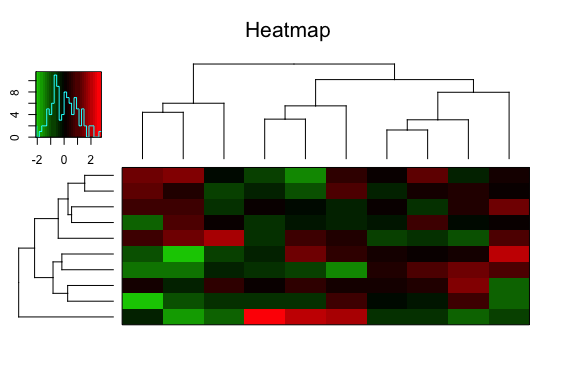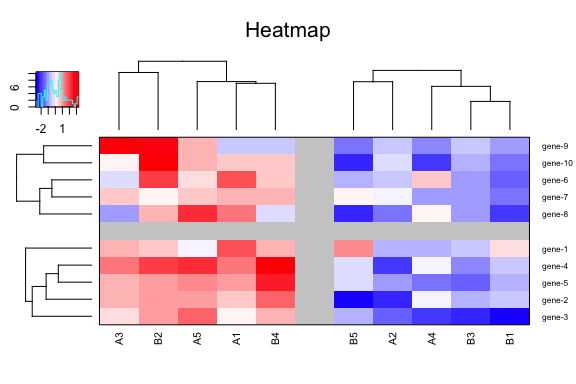Flexible Heatmaps

library(heatmapFlex)
#>
#> Attaching package: 'BiocGenerics'
#> The following objects are masked from 'package:parallel':
#>
#>     clusterApply, clusterApplyLB, clusterCall, clusterEvalQ,
#>     clusterExport, clusterMap, parApply, parCapply, parLapply,
#>     parLapplyLB, parRapply, parSapply, parSapplyLB
#> The following objects are masked from 'package:stats':
#>
#>     IQR, mad, sd, var, xtabs
#> The following objects are masked from 'package:base':
#>
#>     Filter, Find, Map, Position, Reduce, anyDuplicated, append,
#>     as.data.frame, basename, cbind, colnames, dirname, do.call,
#>     duplicated, eval, evalq, get, grep, grepl, intersect, is.unsorted,
#>     lapply, mapply, match, mget, order, paste, pmax, pmax.int, pmin,
#>     pmin.int, rank, rbind, rownames, sapply, setdiff, sort, table,
#>     tapply, union, unique, unsplit, which, which.max, which.min
#> Welcome to Bioconductor
#>
#>     Vignettes contain introductory material; view with
#>     'browseVignettes()'. To cite Bioconductor, see
#>     'citation("Biobase")', and for packages 'citation("pkgname")'.

GPL-3

Description

The package has a number of tools supporting more flexible heatmaps. The graphics is grid-like using the old graphics system. The main function is , which is a wrapper around the various functions constructing individual parts of the heatmap, like sidebars, picket plots, legends etc. The function supports zooming and splitting, i.e., having (unlimited) small heatmaps underneath each other in one plot deriving from the same data set, e.g., clustered and ordered by a supervised clustering method.

Installation

CRAN

install.packages("heatmapFlex")

Latest development version

install.packages("devtools")
devtools::install_github("vfey/heatmapFlex")

Usage

A simple example

Generate a random 10x10 matrix and plot it using default values (which admittedly is not pretty):

mat <- matrix(rnorm(100), nrow = 10)
heatmap.n2(mat)A split heatmap

Generate a random 10x10 matrix with two distinct sets, order it using default clustering methods, split it into each two groups along both rows and columns and adjust colour palette and dendrogram dimensions:

mat <- matrix(c(rnorm(50, mean = 1), rnorm(50, mean = -1)), nrow = 10)
heatmap.n2(mat, col = "BuWtRd", rowMembers=rep(1:2, each=5),
colMembers=rep(1:2, each=5),
labRow=paste0("gene-", 1:10),
labCol=paste0(c("A", "B"), rep(1:5, 2)), r.cex=0.8,
dendroheight = lcm(2.2), dendrowidth = lcm(2.4))A zoomed heatmap

The heatmap is drawn as in the previous example but without splitting. After it has been plotted to a screen graphics device and calling it can be zoomed into by clicking two distinct points inside the plot.

mat <- matrix(c(rnorm(50, mean = 1), rnorm(50, mean = -1)), nrow = 10)
dl <- heatmap.n2(mat, col = "BuWtRd", labRow=paste0("gene-", 1:10),
labCol=paste0(c("A", "B"), rep(1:5, 2)),
r.cex=0.8, dendroheight = lcm(2.2), dendrowidth = lcm(2.4))
zoom_heatmap(dl)